# bodeplot

Plot Bode frequency response with additional plot customization options

## Syntax

```h = bodeplot(sys) bodeplot(sys) bodeplot(sys1,sys2,...) bodeplot(AX,...) bodeplot(..., plotoptions) bodeplot(sys,w) ```

## Description

`h = bodeplot(sys)` plot the Bode magnitude and phase of the dynamic system model `sys` and returns the plot handle `h` to the plot. You can use this handle to customize the plot with the `getoptions` and `setoptions` commands.

`bodeplot(sys)` draws the Bode plot of the model `sys`. The frequency range and number of points are chosen automatically.

`bodeplot(sys1,sys2,...)` graphs the Bode response of multiple models `sys1`,`sys2`,... on a single plot. You can specify a color, line style, and marker for each model, as in

```bodeplot(sys1,'r',sys2,'y--',sys3,'gx') ```

`bodeplot(AX,...)` plots into the axes with handle `AX`.

`bodeplot(..., plotoptions)` plots the Bode response with the options specified in `plotoptions`. Type

```help bodeoptions ```

for a list of available plot options. See Match Phase at Specified Frequency for an example of phase matching using the `PhaseMatchingFreq` and `PhaseMatchingValue` options.

`bodeplot(sys,w)` draws the Bode plot for frequencies specified by `w`. When `w = {wmin,wmax}`, the Bode plot is drawn for frequencies between `wmin` and `wmax` (in `rad/TimeUnit`, where `TimeUnit` is the time units of the input dynamic system, specified in the `TimeUnit` property of `sys`.). When `w` is a user-supplied vector `w` of frequencies, in rad/TimeUnit, the Bode response is drawn for the specified frequencies.

See `logspace` to generate logarithmically spaced frequency vectors.

## Examples

collapse all

Generate a Bode plot.

```sys = rss(5); h = bodeplot(sys);```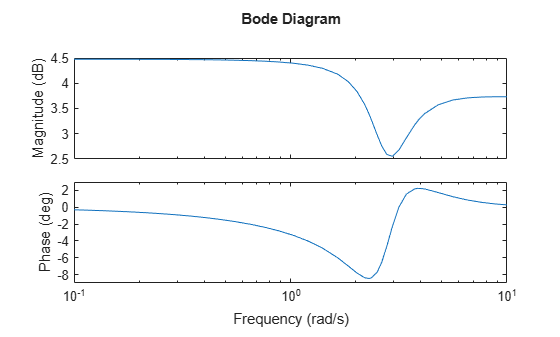Change the units to Hz and suppress the phase plot. To do so, edit properties of the plot handle, `h`.

`setoptions(h,'FreqUnits','Hz','PhaseVisible','off');`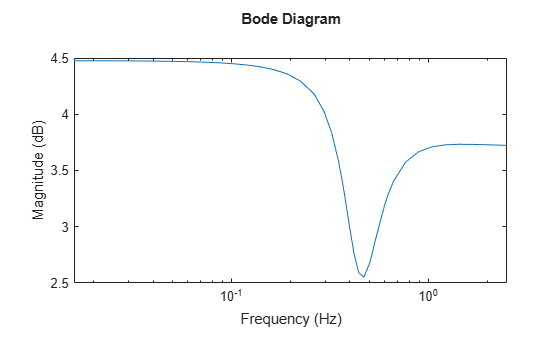The plot automatically updates when you call `setoptions`.

Create a Bode plot of a dynamic system.

```sys = tf(1,[1 1]); h = bodeplot(sys);```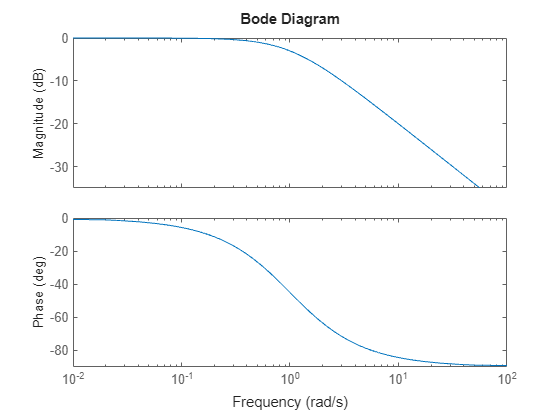Fix the phase at 1 rad/s to 750 degrees. To do so, get the plot properties. Then alter the properties `PhaseMatchingFreq` and `PhaseMatchingValue` to match a phase to a specified frequency.

```p = getoptions(h); p.PhaseMatching = 'on'; p.PhaseMatchingFreq = 1; p.PhaseMatchingValue = 750;```

Update the plot.

`setoptions(h,p);`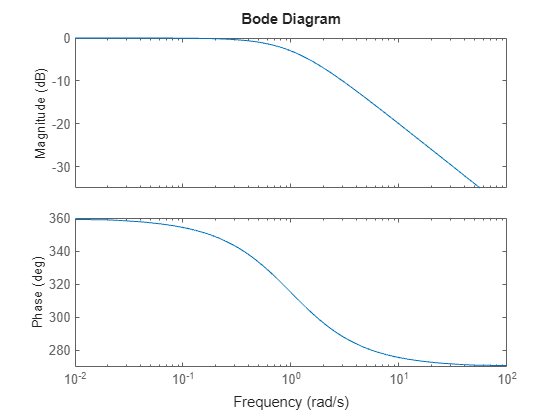The first bode plot has a phase of -45 degrees at a frequency of 1 rad/s. Setting the phase matching options so that at 1 rad/s the phase is near 750 degrees yields the second Bode plot. Note that, however, the phase can only be -45 + N*360, where N is an integer, and so the plot is set to the nearest allowable phase, namely 675 degrees (or 2*360 - 45 = 675).

Compare the frequency responses of identified state-space models of order 2 and 6 along with their 2 $\sigma$ confidence regions.

```load iddata1 sys1 = n4sid(z1, 2); sys2 = n4sid(z1, 6);```

Both models produce about 70% fit to data. However, `sys2` shows higher uncertainty in its frequency response, especially close to Nyquist frequency as shown by the plot:

```w = linspace(8,10*pi,256); h = bodeplot(sys1,sys2,w); setoptions(h,'PhaseMatching','on','ConfidenceRegionNumberSD',2);```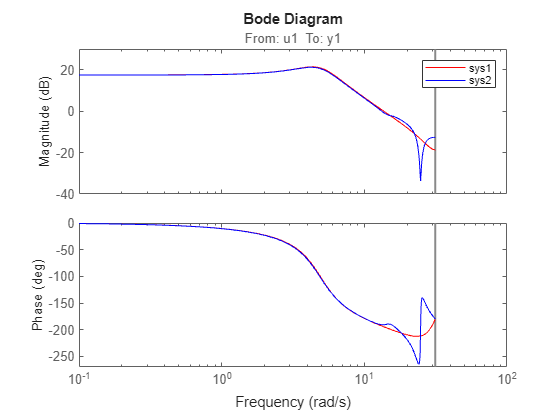Right-click the plot and select Characteristics > Confidence Region to turn on the confidence region characteristic. Alternatively, type `showConfidence(h)` to plot the confidence region.

Compare the frequency response of a parametric model, identified from input/output data, to a nonparametric model identified using the same data. Identify parametric and non-parametric models based on data.

```load iddata2 z2; w = linspace(0,10*pi,128); sys_np = spa(z2,[],w); sys_p = tfest(z2,2);```

`spa` and `tfest` require System Identification Toolbox™ software. `sys_np` is a nonparametric identified model. `sys_p` is a parametric identified model.

Create a Bode plot that includes both systems.

```opt = bodeoptions; opt.PhaseMatching = 'on'; bodeplot(sys_np,sys_p,w,opt);```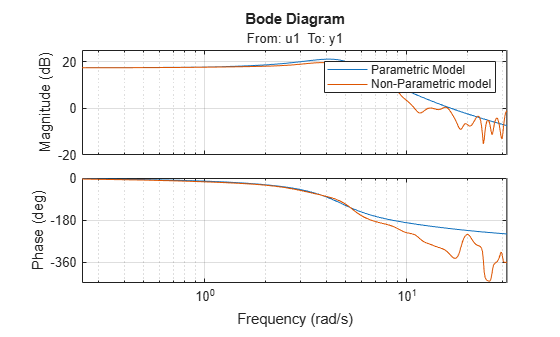## Tips

You can change the properties of your plot, for example the units. For information on the ways to change properties of your plots, see Ways to Customize Plots (Control System Toolbox).